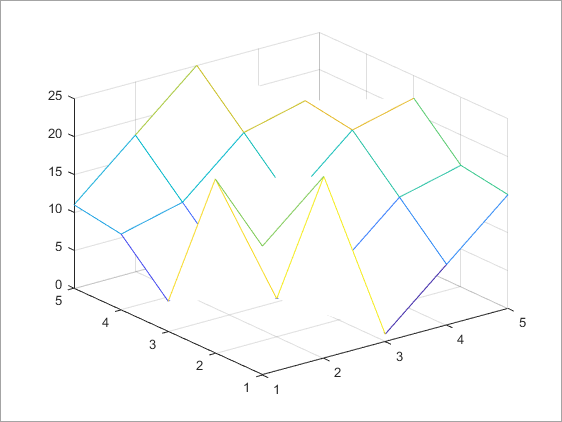# eval

Evaluate MATLAB expression

## Syntax

``eval(expression)``
``[output1,...,outputN] = eval(expression)``

## Description

example

````eval(expression)` evaluates the MATLAB® code in `expression`. NoteSecurity Considerations: When calling `eval` with untrusted user input, validate the input to avoid unexpected code execution. Examples of untrusted user input are data coming from a user you might not know or from a source you have no control over. If you need to address this concern, consider these approaches:Validate inputs to `eval`. First, search for allowed operations. Then, if you find other operations, disallow execution.Replace `eval` with an alternative. For more information, see Alternatives to the eval Function.Performance Considerations: In most cases, using the `eval` function is also less efficient than using other MATLAB functions and language constructs, and the resulting code can be more difficult to read and debug. Consider using an alternative to `eval`. ```

example

````[output1,...,outputN] = eval(expression)` returns the outputs from `expression` in the specified variables.```

## Examples

collapse all

Use `eval` to evaluate and plot the expression `magic(5)`.

```Z = eval('magic(5)'); mesh(Z)```## Input Arguments

collapse all

Expression to evaluate, specified as a character vector or string scalar. `expression` must be a valid MATLAB expression and must not include any MATLAB keywords. To determine whether a word is a MATLAB keyword, use the `iskeyword` function.

Example: `eval('magic(5)')`

## Output Arguments

collapse all

Outputs from evaluated expression, returned as any MATLAB data type.

## Limitations

• If you use `eval` within an anonymous function, nested function, or function that contains a nested function, the evaluated `expression` does not create any variables.

## Tips

• To allow the MATLAB parser to perform stricter checks on your code and avoid untrapped errors and other unexpected behaviors, do not include output arguments in the input to the `eval` function. For example, the statement `eval(['output = ',expression])` is not recommended.

Instead, specify output arguments to the `eval` function to store the results of the evaluated expression. For example:

` output = eval(expression)`

## Version History

Introduced before R2006a